# Continuous Wavelet Technique for Detection of Broken Bar Faults in Induction Machine

Continuous Wavelet Technique for Detection of Broken Bar Faults in Induction Machine
Research Laboratory on the Electrical Engineering, Faculty of Technology, University of M’Sila, 28000, Algeria

Corresponding Author Email:
djalaleddine.khodja@univ-msila.dz
Page:
171-176
|
DOI:
https://doi.org/10.18280/ts.360207
16 January 2019
|
Accepted:
27 March 2019
|
Published:
5 July 2019
| Citation

OPEN ACCESS

Abstract:

The purpose of this study is diagnosis the fault of induction machine, through detection of broken bars by stator current analysis. Therefor we present a multi-winding model for the simulation of faults as part of the fault detection study, and test the behavior of the machine in the healthy and faulty state. In this paper it is focus the signal processing technique for detecting defects. This technique is based on continuous wavelet transform (CWT) to detect and locate defects, by using the multi-level decomposition for the detection of defects and the location of the broken bars at the rotor. The findings of this research results show the importance of this technique for the analysis of fault signatures in healthy and defective cases.

Keywords:

continuous wavelet (CWT), induction machine diagnosis, signal processing, faults signatures, indicator values

1. Introduction

The increasing use of induction machines in electric drive systems plays a major role in the industrial field such as, Safety, reliability, efficiency and performance, the importance of failure detection is often required in order to improve performance and reduce maintenance costs to avoid a sudden stop of the machine. On the other hand, the diagnostic role is the identification and location of defects [1-2]. In this context, the proposed approach is examined on three different cases of study : a motor with broken rotor bars, an unbalanced motor shaft, based on the startup vibration transient analysis. by uses signal processing based on technique continuous wavelet CWT. Whilst others [4-5] to introduce technique (MCSA) motor current signature analysis which utilizes the results of spectral analysis of the stator current for fault detection and the localization of abnormal electrical and mechanical conditions that indicate, a failure of induction motors. Among the diagnoses of cage rotor faults in three-phase asynchronous machines, the parks current vector monitoring technique by , for detect the occurrence of cage rotor fractures. The detection of this type of defects can be easily obtained by observing at the vector representation of the current of the motor Park. The detection fault in induction motor is most explored because when this fault the motor keeps working with occurs appear noise and vibration. Therefore Most of the proposed techniques single processing is based on analysis of the stator currents, by using fast Fourier transform (FFT) and wavelet.

Among the works for broken bar detection is the work of [7-8] presents role Wavelet Transform (WT) for analysis the startup transient the current signals of squirrel cage induction motor with broken rotor bars . Who proposes failure predictions in three phase line-operated induction machines through spectral analysis of electric and electromagnetic signals, through frequency signature of some asymmetrical motor faults . Present an algorithm for the online detection of rotor bar breakage in induction motors through the use of wavelet packet decomposition (WPD) and neural networks used by motor stator current, for extracted features with different frequency resolutions.  focuses on the study of an approximation signal resulting from the wavelet decomposition of the start-up stator current using neuro fuzzy logic for find the rotor bar failures.

This work is divided into three main sections, firstly we described several types fault found in machine and presented Multi-winding model for simulate and study behavior of asynchronous machines in case healthy and faults with broken bars. Secondly, it focuses on role technique single processing based on continuous wavelet which is very well adapted to study the characteristics of the fault signals. Finally we will finish by presenting the results of the fault signatures simulation and application of continuous wavelet technique (CWT) in order to detect and identify the type of defects.

2. Detection of Several Faults in Induction Machine

2.1 Broken bars

The rotor defect now accounts for about 5 % to 10 % of total rotor failure. This defect induces changes in the stator currents and therefore causes the appearance of characteristic harmonics in the spectrum of this signal. Indeed, during the failure of a bar break, flux harmonics are produced and induce current harmonics in the stator winding at frequencies around the fundamental frequency fs for detection broken bar using components spectral the current stator these components spectral given by relation :

fbrb=fs (1±2ks) f    (1)

g: Slip.

fs: Stator power frequency.

k = 1.2.3……

Figure 1 shows the four adjacent bar breaks used for the simulation study.

## f1.pngFigure 1. Picture show the two bar broken adjacent

2.2 Bearing faults

Detecting bearing he have the rate 40-50 % of induction motors faults This type of defect will produce one of the four characteristic fault frequencies in the machine vibration depending on which bearing surface contains the fault. Most electrical machines use either ball or rolling-element bearing [13-14]. This frequency is illustrated in Figure 2.

## f2.png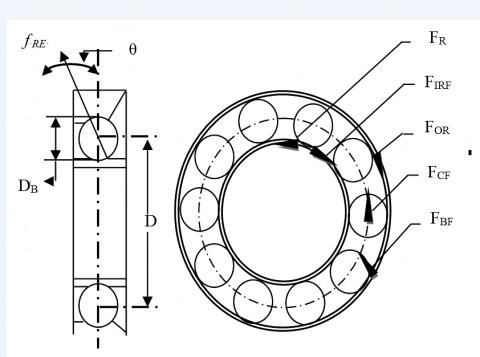Figure 2. Dimensions and frequencies related to the characteristics bearing faults

2.3 Air gap eccentricity

Air gap between stator and rotor results in eccentricity of induction motor, air gap eccentricity can be divides tow type: the static and dynamic of air-gap eccentricity. where static eccentricity when the position of minimum radial length of the air-gap is fixed in the space, in this case the rotor axis coincides with the shaft axis, but does not coincide with the stator axis. Further for dynamic eccentricity when the position of minimum radial length of the air-gap rotates with the rotor, in this case the shaft axis does not coincide with the rotor axis (the rotor does not rotate about its axis) [15-16]. This air gap eccentricity static and dynamic is illustrated in Figure 3:

## f3.png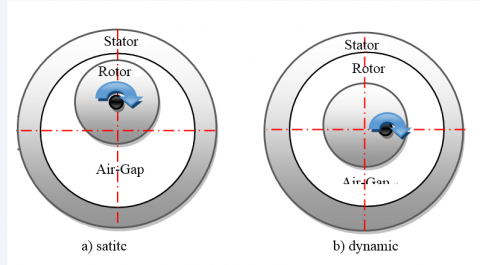Figure 3. Air gap eccentricity illustrate tow type static and dynamic

3. Continuous Wavelet Transform

The wavelets are constructed from a “mother wavelet”$\psi$ ϵ L2 (R) using a translation and expansion parameters continuously vary this time translation operator and a change of scale operator the transform used the functions [17-18]:

$\Psi_{(a, b)}(X)=\frac{1}{\sqrt{a}} \psi\left(\frac{X-b}{a}\right)$  (2)

With: a, b ϵ R

a: Scale factor

b: Translate or space.

x: He distance is used to expand (compress or extend) the function $\psi$.

The wavelet continuous CWT is the technique used in this article for detection the faults.

4. Multi-Winding Modele Mathematique of the Asynchronous Machine

The application of extended Park transformation of rotor system so as to transform the system in Nr phases in a system (d,q). The mathematical model of squirrel cage induction motor can be written as:

$[L] \frac{d I}{d t}=[V]-[R][I]$   (3)

For Defect model of the rotor to simulate of rotor broken bars, a fault resistance RRF is added to the corresponding element of the rotor resistance matrix RR [19-20]:

$\left[\mathrm{R}_{\mathrm{RF}}\right]_{\mathrm{N}_{\mathrm{R}} \mathrm{xN}_{\mathrm{R}}}=\left[\mathrm{R}_{\mathrm{R}}\right]_{\mathrm{N}_{\mathrm{R}} \mathrm{xN}_{\mathrm{R}}}-\left[\mathrm{R}_{\mathrm{F}}\right]_{\mathrm{N}_{\mathrm{R}} \mathrm{xN}_{\mathrm{R}}}$   (4)

$\left[R_{R F}\right]=\left[\mathrm{R}_{R}\right]+\left[\begin{array}{cccccccc}{0} & {\cdots} & {0} & {0} & {0} & {\cdots} & {\cdots} & {\cdots} \\ {\vdots} & {\cdots} & {\vdots} & {\vdots} & {\vdots} & {\vdots} & {\cdots} \\ {0} & {\cdots} & {0} & {0} & {0} & {0} & {\cdots} \\ {0} & {\ldots} & {0} & {R_{b k}} & {-R_{b k}} & {0} & {\cdots} \\ {0} & {\cdots} & {0} & {-R_{b k}} & {R_{b k}} & {0} & {\cdots} \\ {0} & {\cdots} & {0} & {0} & {0} & {0} & {\cdots} \\ {\vdots} & {\cdots} & {\vdots} & {\vdots} & {\vdots} & {\vdots} & {\ldots}\end{array}\right]$

The new matrix of rotor resistances, after transformations, becomes:

$\left[R_{R F}\right]=\left[\begin{array}{cc}{R_{r d d}} & {R_{r d q}} \\ {R_{r q d}} & {R_{r q q}}\end{array}\right]$

where the four terms of this matrix are:

$R_{r d d}=2 R_{b}(1-\cos (a))+2 \frac{R_{e}}{N_{r}}+\frac{2}{N_{r}}(1-\cos (a) ) \sum_{k} R_{b k f}(1-\cos (2 k-1) a)$   (5)

$R_{r d q}=-\frac{2}{N_{r}}(1-\cos (a)) \sum_{k} R_{b k f} \sin (2 k-1) a )$   (6)

$R_{r q d}=-\frac{2}{N_{r}}(1-\cos (a)) \sum_{k} R_{b k f} \sin (2 k-1) a$   (7)

$\operatorname{R_{rqq}}=2 R_{b}(1-\cos (a))+2 \frac{R_{e}}{N_{r}}+\frac{2}{N_{r}}(1-\cos (a) ) \sum_{k} R_{b k f}(1+\cos (2 k-1) a)$   (8)

Finally. equation model the fault of induction motor can be written as: $[\mathrm{L}] \frac{\mathrm{d} \mathrm{I}}{\mathrm{dt}}=[\mathrm{V}]-[\mathrm{R}][\mathrm{I}]$

$\left[\begin{array}{ccccc}{\mathrm{L}_{\mathrm{sc}}} & {0} & {-\mathrm{N}_{\mathrm{r}} \mathrm{M}_{\mathrm{sr}} / 2} & {0} & {0} \\ {0} & {\mathrm{L}_{\mathrm{sc}}} & {0} & {\mathrm{N}_{\mathrm{r}} \mathrm{M}_{\mathrm{sr}} / 2} & {0} \\ {-3 \mathrm{M}_{\mathrm{sr}} / 2} & {0} & {\mathrm{L}_{\mathrm{rc}}} & {0} & {0} \\ {0} & {3 \mathrm{M}_{\mathrm{sr}} / 2} & {0} & {\mathrm{L}_{\mathrm{rc}}} & {0} \\ {0} & {0} & {0} & {0} & {\mathrm{L}_{\mathrm{e}}}\end{array}\right] \frac{\mathrm{d}}{\mathrm{d} \mathrm{t}}\left[\begin{array}{c}{\mathrm{I}_{\mathrm{ds}}} \\ {\mathrm{I}_{\mathrm{qs}}} \\ {\mathrm{I}_{\mathrm{dr}}} \\ {\mathrm{I}_{\mathrm{qr}}} \\ {\mathrm{I}_{\mathrm{e}}}\end{array}\right]=\left[\begin{array}{c}{\mathrm{V}_{\mathrm{ds}}} \\ {\mathrm{V}_{\mathrm{q}}} \\ {0} \\ {0} \\ {0}\end{array}\right]$

$-\left[\begin{array}{ccccc}{\mathrm{R}_{\mathrm{s}}} & {-\mathrm{L}_{\mathrm{sc}} \omega_{\mathrm{r}}} & {0} & {\mathrm{N}_{\mathrm{r}} \mathrm{M}_{\mathrm{sr}} \omega_{\mathrm{r}} / 2} & {0} \\ {\mathrm{L}_{\mathrm{sc}} \omega_{\mathrm{r}}} & {\mathrm{R}_{\mathrm{s}}} & {\mathrm{N}_{\mathrm{r}} \mathrm{M}_{\mathrm{sr}} \omega_{\mathrm{r}} / 2} & {0} & {0} \\ {0} & {0} & {\mathrm{R}_{\mathrm{r}}} & {0} & {0} \\ {0} & {0} & {0} & {\mathrm{R}_{\mathrm{r}}} & {0} \\ {0} & {0} & {0} & {0} & {\mathrm{R}_{\mathrm{e}}}\end{array}\right]\left[\begin{array}{c}{\mathrm{I}_{\mathrm{ds}}} \\ {\mathrm{I}_{\mathrm{qs}}} \\ {\mathrm{I}_{\mathrm{dr}}} \\ {\mathrm{I}_{\mathrm{qr}}} \\ {\mathrm{I}_{\mathrm{e}}}\end{array}\right]$

where:

$\left[R_{R F}\right]=\left[\mathrm{R}_{R}\right]+\left[\begin{array}{cccccccc}{0} & {\cdots} & {0} & {0} & {0} & {\cdots} & {\cdots} & {\cdots} \\ {\vdots} & {\cdots} & {\vdots} & {\vdots} & {\vdots} & {\vdots} & {\cdots} \\ {0} & {\cdots} & {0} & {0} & {0} & {0} & {\cdots} \\ {0} & {\ldots} & {0} & {R_{b k}} & {-R_{b k}} & {0} & {\cdots} \\ {0} & {\cdots} & {0} & {-R_{b k}} & {R_{b k}} & {0} & {\cdots} \\ {0} & {\cdots} & {0} & {0} & {0} & {0} & {\cdots} \\ {\vdots} & {\cdots} & {\vdots} & {\vdots} & {\vdots} & {\vdots} & {\ldots}\end{array}\right]$

And

$[L]=\left[\begin{array}{ccccc}{L_{s c}} & {0} & {-N_{r} M_{s r} / 2} & {0} & {0} \\ {0} & {L_{s c}} & {0} & {N_{r} M_{s r} / 2} & {0} \\ {-3 M_{s r} / 2} & {0} & {L_{r c}} & {0} & {0} \\ {0} & {-3 M_{s r} / 2} & {0} & {L_{r c}} & {0} \\ {0} & {0} & {0} & {0} & {L_{e}}\end{array}\right]$

$L_{r c}=L_{r p}-M_{r r}+\frac{2 L_{e}}{N_{r}}+2 L_{e}(1-\cos (a))$   (9)

and

$R_{r}=\frac{2 R_{e}}{N_{r}}+2 R b(1-\cos (a))$   (10)

The mechanical equations must also consider:

$\frac{d}{d t} \omega=\frac{1}{j}\left(C_{e}-C_{r}\right)$   (11)

With:

$\omega=\frac{d \theta}{d t}$

The electromagnetic torque with the expression:

$C_{e}=\frac{3}{2} p \cdot N_{r} \cdot M_{s r}\left(I_{d s} \cdot I_{q r}-I_{q s} \cdot I_{d r}\right)$   (12)

For observe and simulate the multi-winding mode four study the influence of broken bar faults and analyze type of the components which occurs when appear defect in asynchronous machine. Figure 4, 5 and 6, show the evolution of the electromagnetic torque and the speed of rotation and the stator current, at time 0.5 s apply torque of resistor 3.5 (N.m) in case of healthy, the curve speed rises rapidly then reaches a peak and then forms a plateau without wavy, the curve electromagnetic there was a sudden increaseat t=0.5 s, then after that Stabilizes, the curve stator current Stabilizes without wavy. In case of fault machine, the increase of the rotor bar resistance creates the proportional defects with number of broken bars. We also notice the four successive broken bars, the first at time t=1s, the second at time t=2s, and then a third at time t=3s, and fourth time t=4s. In the following Figures we notice the Figure 7 of rotational speed decreases and increase the undulations after the breaking four bar adjacent. Figure 8 of electromagnetic torque increase in the undulations, the Figure 9 are illustrated modulation of the envelope of the stator current after broken bar is proportional the number of break baradjacent.

## f4.png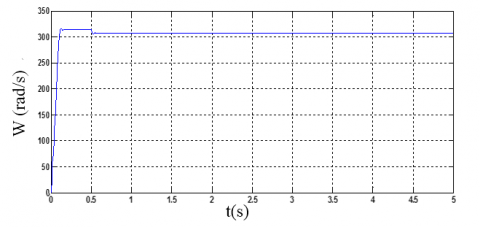Figure 4. Speed for the healthy machine

## f5.png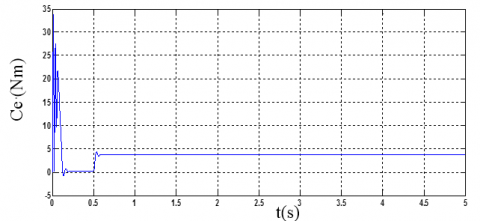Figure 5. Electromagnetic torque for the healthy machine

## f6.png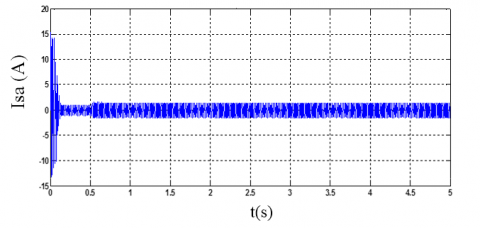Figure 6. Stator current for the healthy machine

## f7.pngFigure 7. Rotation speed with 4 broken bars

## f8.pngFigure 8. Torque with 4 broken bars

## f9.png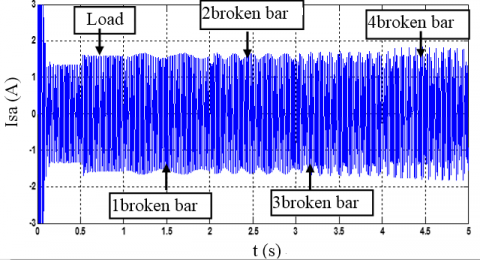Figure 9. Stator current with 4 broken bars

The figures illustrate the application of the continuous wavelet transform of the stator current of a phase (a) using the continuous wavelet transform (CWT), for the scales between 0 and 64 respectively and Meyer wavelet family in the case: healthy and defect (breakage of other adjacent bars at time t=1s, t=2s, t=3s, t=4s show, transformed into continuous wavelet (CWT) shows that wavelet analysis is a measure of similarity between basic functions (wavelets) and the signal itself, the similarity is in the sense of the same frequency content The calculated coefficients of (CWT) refer to the proximity of the signal to the wavelet at the current scale. a, b) in the case of a healthy state, no change in the current signal is stable, but in the case of defects (breaking one bar and two bar, three bar, four adjacent bar, a change in the coefficient can be observed in the figures (10-11-12-13-14), where the wave coefficients the motor fault are stronger than the wavelet coefficients of the healthy motor. These differences indicate that the wavelet transformation is able to detect changes in the signal components between the failed motor and the healthy motor during the start-up period. for High scales corresponds to low frequencies. and the low scales correspond to the high frequencies. As a result, the small peak of the graph corresponds to high frequency components and the broad peak corresponding to low frequency components of the signal (which appear in time before the high frequency components). The table shows the peak of the scales using analysis of the stator current by continuous wavelet (CWT).

Table 1. Shows the peak of the scales by (CWT)

 Type faults Broken one bar Broken tow bar Broken three bar Broken four bar Peak of the scales 23.4 24.2 27.1 31.3

Absolute Values of Ca,b Coefficients for a = 1 2 3 4 5 ...

## f10.png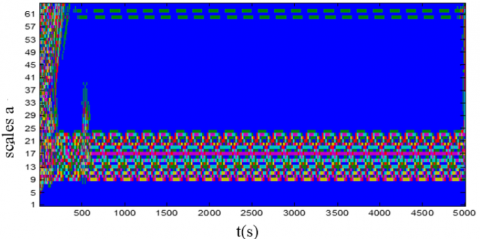Figure 10. Stator current using Wavelet (CWT) in healthy case

Absolute Values of Ca,b Coefficients for a = 1 2 3 4 5 ...

## f11.png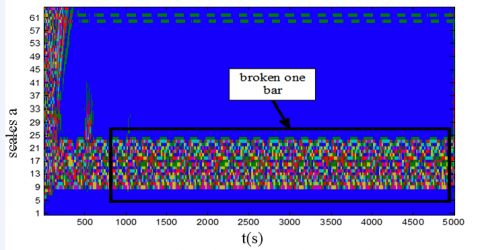Figure 11. Stator current using Wavelet (CWT) in case broken one bar

Absolute Values of Ca,b Coefficients for a = 1 2 3 4 5 ...

## f12.pngFigure 12. Stator current using Wavelet (CWT) in case broken two bar

Absolute Values of Ca,b Coefficients for a = 1 2 3 4 5 ...

## f13.pngFigure 13. Stator current using Wavelet (CWT) in case broken three bar

Absolute Values of Ca,b Coefficients for a = 1 2 3 4 5 ...

## f14.pngFigure 14. Stator current using Wavelet (CWT) in case broken four bar

5. Conclusions

The main objective of our work is to detect and diagnose defect in the asynchronous machine through the use of a multi-winding model for simulate the break of bars and determine the behaviour of the machine in the state of healthy and defective. The increasing the breaking bars inevitably lead to deterioration of the performance and effectiveness of the machine. The advantage of the use of the signal processing allows the extraction of the information to identify and locate the defects. Also, to guarantee an operating without stop of the machine based on the use of the technique wavelet continuous transformation. the obtained simulation results show the role of this technique for detection broken bars. Further to this, to improve the fault detection, it can be used by the wavelet kind quality, to get elevated raise from detection and diagnostics effectiveness. This method is certain technology for condition monitoring and fault analysis of motors, and detects these problems at an early period and thus avoid damage and complete failure. The shortened time is guaranteed to identify malfunctions and ensure maintenance of the machine without damaging any part of the machine.

Acknowledgment

This work was supported by Electrical Engineering Laboratory (LGE), at the University of Mohamed Boudiaf- M’sila, (Algeria). We like to thanks Dr. Djalal Eddine Khodja, for their helps in the preparing of this paper.

Appendix

Table 2. Appendix

 Symbol Definition Value Pn Output power 1.1 kW Vs Stator voltage per phase 220 V Fs Stator frequency 50 Hz p Poles pair number 1 Rs Stator resistance 7.58 Ω Rr Rotor resistance 6.3 Ω Rb Rotor bar resistance 0.15 m Ω Re Resistance of end ring segment 0.15 m Ω Lb Rotor bar inductance 0.1 μH Le Inductance of end ring 0.1 μH Lsf Leakage inductance of stator 0.0265 H Nr Number of rotor bars 16 Ns Number of turns per stator phase 160 J Inertia moment 0.0054 kg m2 e Air-gap mean diameter 2 mm

References

 Ma, M.Y., Hu, L., Chen, A., He, X.N. (2007). Reconfiguration of carrier-based modulation strategy for fault tolerant multilevel inverters. IEEE Transactions on Power Electronics, 22(5): 2050-2060. https://doi.org/10.1109/TPEL.2007.904249

 Aguayo, J., Claudio, A., Vela, L., Gentile, S. (2005). A survey of fault diagnosis methods for induction motors drives under inverter fault conditions. International Conference of Electrical and Electronics Engineering, (ICEEE). Acapulco Guerrero, México, pp. 367-372. https://doi.org/10.1109/ICEEE.2004.1433910

 Rodriguez-Donate, C., Romero-Troncoso, R.J., Cabal, E.Y., Garcia-Perez, A. (2017). Wavelet-based general methodology for multiple fault detection on induction motors at the startup vibration transient. Journal of Vibration and Control, 17(9): 1299-1309. https://doi.org/10.1177/1077546310379141

 Chaitali, S., Kalaskar, V. (2014). Motor current signature analysis to detect the fault in induction motor. Journal of Engineering Research and Applications, 4(6): 58-61.

 Cusido, J., Garcia, J.R., Aldabas, E., Antonio Ortege, J., Romeral, L. (2006). New fault detection techniques for induction motors. Electrical Power Quality and Utilisation Magazine, 11(1): 39-46. https://doi.org/10.1109/CPE.2005.1547550

 Costa, C.D., Kashiwagi, M., Mathias, M.H. (2015). Rotor failure diagnosis of induction motors by wavelet transformand fourier transform in function of the load. 1(15): 15-26.

 Anik, V. (2017). Fault diagnosis of induction motor using wavelets. International Journal of Research and Engineering, 4(4): 125-126.

 Gaeid Salloum, K., Ping, H.W. (2011). Wavelet fault diagnosis and tolerant of induction motor: A review. International Journal of the Physical Sciences, 6(3): 358-376. https://doi.org/10.5897/IJPS10.632

 Eltabach, M., Charara, A., Zein, I. (2004). A comparison of external and internal methods of signal spectral analysis for broken rotor bars detection in induction motors. IEEE Transactions on Industry Applications, 51(1): 107-121. https://doi.org/10.1109/TIE.2003.822083

 Sadeghian, A., Ye, Z.M., Wu, B. (2009). Online detection of broken rotor bars in induction motors by wavelet packet decomposition and artificial neural networks. IEEE Transactions on instrumentation and Measurement, 58(7): 2253-2263. https://doi.org/10.1109/TIM.2009.2013743

 Chandralekha, R., Jayanthi, D. (2016). Diagnosis of faults in three phase induction motor using neuro fuzzy logic. International Journal of Applied Engineering Research, 11(8): 5735-5740.

 Yang, T., Pen, H.B., Wang, Z.X. Chang, C.S. (2016). Feature knowledge based fault detection of induction motors through the analysis of stator current data. IEEE Transactions on instrumentation and Measurement, 65(3): 549-558. https://doi.org/10.1109/TIM.2015.2498978

 Cruz, S.M.A., Cardoso, A.J.M. (2014). Rotor cage fault diagnosis in three-phase induction motors by extended park's vector approach. Electric Machines & Power Systems, 28(4): 289-299. https://doi.org/10.1080/073135600268261

 Cruz, S.M.A., Cardoso, A.J.M. (2000). Stator winding fault diagnosis in three-phase synchronous and asynchronous motors, by the extended park’s vector approach. IEEE Transactions on Industry Applications, 37(5): 1227-1233. https://doi.org/10.1109/28.952496

 Cardoso, A.J.M., Cruz, S.M.A., Carvalho, J.F.S., Saraiva, E.S. (1995). Rotor cage fault diagnosis in three-phase induction motors, by park's vector approach. Proceedings of the 1995 IEEE, San Diego (USA), pp. 642-646. https://doi.org/10.1109/IAS.1995.530360

 Filippetti, F., Franceschini, G., Tassoni, C., Vas, P. (1994). Broken bar detection in induction machines: Comparison between current spectrum approach and parameter estimation approach. Industry Applications Society Annual Meeting, Conference Record of the IEEE, pp. 95-102. https://doi.org/10.1109/IAS.1994.345493

 Ahamed, S.K., Sarkar, A., Madhuchhanda, M., Sengupta, S. (2012). Detection of induction motor broken bar fault through envelope analysis using start-up current. Procedia Technology, 4: 646-651. https://doi.org/10.1016/j.protcy.2012.05.104

 Watson, S.J, Xiang, B.J., Yang, W.X., Tavner, P.J. (2010). Condition monitoring of the power output of wind turbine generators using wavelets. IEEE transactions on energy conversion, 25(3): 715-721. https://doi.org/10.1109/TEC.2010.2040083

 Menacer, A., Benakcha, A., Nait Said, M.S., Drid, S. (2004). Stator current analysis of incipient fault into asynchronous motor rotor bars using fourier fast transform. Journal of Electrical Engineering, 55(6): 122-130.

 Nemec, M., Ambrožič, V., Fišer, R., Nedeljković, D., Drobnič, K. (2019). Induction motor broken rotor bar detection based on rotor flux angle monitoring. Energies, 12(5): 794-811. https://doi.org/10.3390/en12050794